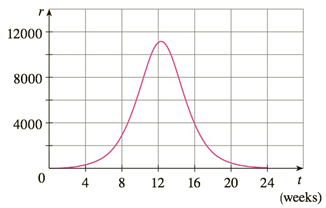Chapter 4.R, Problem 49E

Chapter
Section
Textbook Problem

# A population of honeybees increased at a rate of r ( t ) bees per week, where the graph of r is as shown. Use the Midpoint Rule with six subintervals to estimate the increase in the bee population during the first 24 weeks.To determine

To estimate:

The increase in the bee population during the first 24 weeks

Explanation

1) Concept:

i) The midpoint rule: abfxdxi=1nf(xi-)x=x[fx1-+·····+fxn-]

Where x=b-an  and  xi-=12xi-1+xi= midpoint of [xi-1,xi] where xi=a+ix

2) Calculation:

Apply the midpoint rule for the given values

abfxdxi=1nf(xi-)x=x[fx1-+·····+fxn-]  Where x=b-an  and  xi-=12xi-1+xi= midpoint of [xi-1,x

### Still sussing out bartleby?

Check out a sample textbook solution.

See a sample solution

#### The Solution to Your Study Problems

Bartleby provides explanations to thousands of textbook problems written by our experts, many with advanced degrees!

Get Started